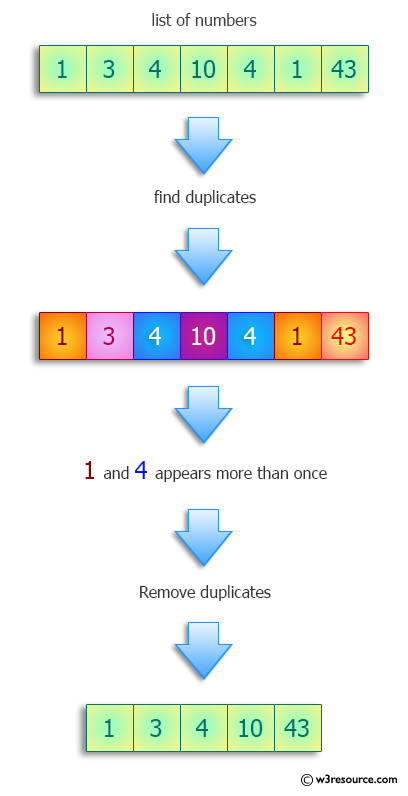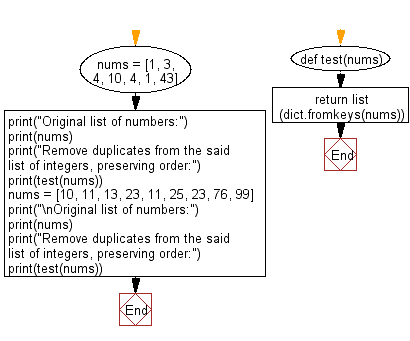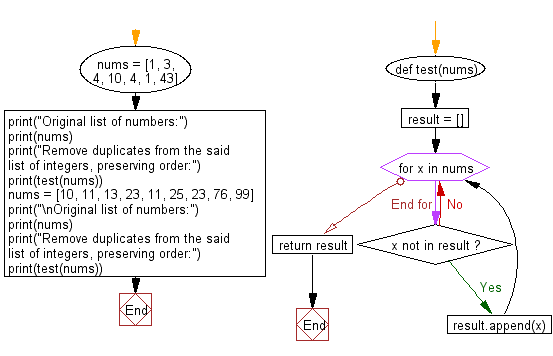﻿ Python: Remove duplicates from a list of integers, preserving order - w3resource# Python: Remove duplicates from a list of integers, preserving order

## Python Programming Puzzles: Exercise-54 with Solution

Write a Python program to remove duplicates from a list of integers, preserving order.

```Input:
[1, 3, 4, 10, 4, 1, 43]
Output:
[1, 3, 4, 10, 43]

Input:
[10, 11, 13, 23, 11, 25, 23, 76, 99]
Output:
[10, 11, 13, 23, 25, 76, 99]
```

Pictorial Presentation:Sample Solution-1:

Python Code:

``````#License: https://bit.ly/3oLErEI

def test(nums):
return list(dict.fromkeys(nums))
nums = [1, 3, 4, 10, 4, 1, 43]
print("Original list of numbers:")
print(nums)
print("Remove duplicates from the said list of integers, preserving order:")
print(test(nums))
nums = [10, 11, 13, 23, 11, 25, 23, 76, 99]
print("\nOriginal list of numbers:")
print(nums)
print("Remove duplicates from the said list of integers, preserving order:")
print(test(nums))
``````

Sample Output:

```Original list of numbers:
[1, 3, 4, 10, 4, 1, 43]
Remove duplicates from the said list of integers, preserving order:
[1, 3, 4, 10, 43]

Original list of numbers:
[10, 11, 13, 23, 11, 25, 23, 76, 99]
Remove duplicates from the said list of integers, preserving order:
[10, 11, 13, 23, 25, 76, 99]
```

Flowchart:## Visualize Python code execution:

The following tool visualize what the computer is doing step-by-step as it executes the said program:

Sample Solution-2:

Python Code:

``````#License: https://bit.ly/3oLErEI

def test(nums):
result = []
for x in nums:
if x not in result:
result.append(x)
return result

nums = [1, 3, 4, 10, 4, 1, 43]
print("Original list of numbers:")
print(nums)
print("Remove duplicates from the said list of integers, preserving order:")
print(test(nums))
nums = [10, 11, 13, 23, 11, 25, 23, 76, 99]
print("\nOriginal list of numbers:")
print(nums)
print("Remove duplicates from the said list of integers, preserving order:")
print(test(nums))
``````

Sample Output:

```Original list of numbers:
[1, 3, 4, 10, 4, 1, 43]
Remove duplicates from the said list of integers, preserving order:
[1, 3, 4, 10, 43]

Original list of numbers:
[10, 11, 13, 23, 11, 25, 23, 76, 99]
Remove duplicates from the said list of integers, preserving order:
[10, 11, 13, 23, 25, 76, 99]
```

Flowchart:## Visualize Python code execution:

The following tool visualize what the computer is doing step-by-step as it executes the said program:

Python Code Editor :

Have another way to solve this solution? Contribute your code (and comments) through Disqus.

What is the difficulty level of this exercise?

Test your Programming skills with w3resource's quiz.

﻿

## Python: Tips of the Day

Clamps num within the inclusive range specified by the boundary values x and y:

Example:

```def tips_clamp_num(num,x,y):
return max(min(num, max(x, y)), min(x, y))
print(tips_clamp_num(2, 4, 6))
print(tips_clamp_num(1, -1, -6))
```

Output:

```4
-1
```